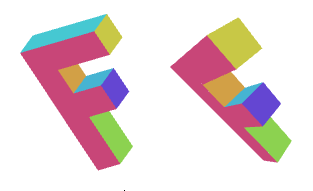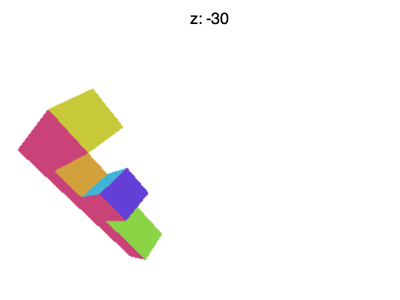# WebGLFundamentals.org

Fix, Fork, Contribute

# WebGL 三维透视投影```10 / 1 = 10
20 / 1 = 20
abs(10-20) = 10
```

```10 / 2 = 5
20 / 2 = 10
abs(5 - 10) = 5
```

```10 / 3 = 3.333
20 / 3 = 6.666
abs(3.333 - 6.666) = 3.333
```

``````<script id="vertex-shader-3d" type="x-shader/x-vertex">
...
+uniform float u_fudgeFactor;
...
void main() {
// 将位置和矩阵相乘
vec4 position = u_matrix * a_position;

+  // 调整除数
+  float zToDivideBy = 1.0 + position.z * u_fudgeFactor;

*  // x 和 y 除以调整后的除数
*  gl_Position = vec4(position.xy / zToDivideBy, position.zw);
}
</script>
``````

``````  ...
var fudgeLocation = gl.getUniformLocation(program, "u_fudgeFactor");

...
var fudgeFactor = 1;
...
function drawScene() {
...
// 设置 fudgeFactor
gl.uniform1f(fudgeLocation, fudgeFactor);

// 绘制几何体
var primitiveType = gl.TRIANGLES;
var offset = 0;
var count = 16 * 6;
gl.drawArrays(primitiveType, offset, count);
``````orthographic vs perspective

``````<script id="vertex-shader-2d" type="x-shader/x-vertex">
...
uniform float u_fudgeFactor;
...
void main() {
// 将位置和矩阵相乘
vec4 position = u_matrix * a_position;

// 调整除数
float zToDivideBy = 1.0 + position.z * u_fudgeFactor;

// 将 x y z 除以 zToDivideBy
*  gl_Position = vec4(position.xyz, zToDivideBy);

// 传递颜色到给片段着色器
v_color = a_color;
}
</script>
``````

```1, 0, 0, 0,
0, 1, 0, 0,
0, 0, 1, 1,
0, 0, 0, 0,
```

```x_out = x_in * 1 +
y_in * 0 +
z_in * 0 +
w_in * 0 ;

y_out = x_in * 0 +
y_in * 1 +
z_in * 0 +
w_in * 0 ;

z_out = x_in * 0 +
y_in * 0 +
z_in * 1 +
w_in * 0 ;

w_out = x_in * 0 +
y_in * 0 +
z_in * 1 +
w_in * 0 ;
```

```x_out = x_in;
y_out = y_in;
z_out = z_in;
w_out = z_in;
```

```1, 0, 0, 0,
0, 1, 0, 0,
0, 0, 1, 1,
0, 0, 0, 1,
```

```w_out = x_in * 0 +
y_in * 0 +
z_in * 1 +
w_in * 1 ;
```

```w_out = z_in + 1;
```

```1, 0, 0, 0,
0, 1, 0, 0,
0, 0, 1, fudgeFactor,
0, 0, 0, 1,
```

```w_out = x_in * 0 +
y_in * 0 +
z_in * fudgeFactor +
w_in * 1 ;
```

```w_out = z_in * fudgeFactor + 1;
```

``````<script id="vertex-shader-2d" type="x-shader/x-vertex">
uniform mat4 u_matrix;

void main() {
// 位置和矩阵相乘
gl_Position = u_matrix * a_position;
...
}
</script>
``````

``````function makeZToWMatrix(fudgeFactor) {
return [
1, 0, 0, 0,
0, 1, 0, 0,
0, 0, 1, fudgeFactor,
0, 0, 0, 1,
];
}
``````

``````    ...
// 计算矩阵
*    var matrix = makeZToWMatrix(fudgeFactor);
*    matrix = m4.multiply(matrix, m4.projection(gl.canvas.clientWidth, gl.canvas.clientHeight, 400));
matrix = m4.translate(matrix, translation, translation, translation);
matrix = m4.xRotate(matrix, rotation);
matrix = m4.yRotate(matrix, rotation);
matrix = m4.zRotate(matrix, rotation);
matrix = m4.scale(matrix, scale, scale, scale);

...
````````````var m4 = {
perspective: function(fieldOfViewInRadians, aspect, near, far) {
var f = Math.tan(Math.PI * 0.5 - 0.5 * fieldOfViewInRadians);
var rangeInv = 1.0 / (near - far);

return [
f / aspect, 0, 0, 0,
0, f, 0, 0,
0, 0, (near + far) * rangeInv, -1,
0, 0, near * far * rangeInv * 2, 0
];
},

...
``````

``````var aspect = gl.canvas.clientWidth / gl.canvas.clientHeight;
var zNear = 1;
var zFar = 2000;
var matrix = m4.perspective(fieldOfViewRadians, aspect, zNear, zFar);
matrix = m4.translate(matrix, translation, translation, translation);
matrix = m4.xRotate(matrix, rotation);
matrix = m4.yRotate(matrix, rotation);
matrix = m4.zRotate(matrix, rotation);
matrix = m4.scale(matrix, scale, scale, scale);
``````

### 为什么将 F 移动到那么远的距离(Z = -360)?

• 基础概念
• 图像处理
• 二维平移，旋转，缩放和矩阵运算
• 三维
• 光照
• 组织和重构
• 几何
• 纹理
• 渲染到纹理
• 阴影
• 技术
• 建议
• 优化
• 杂项
• 参考

Issue/Bug? 在GitHub上提issue.